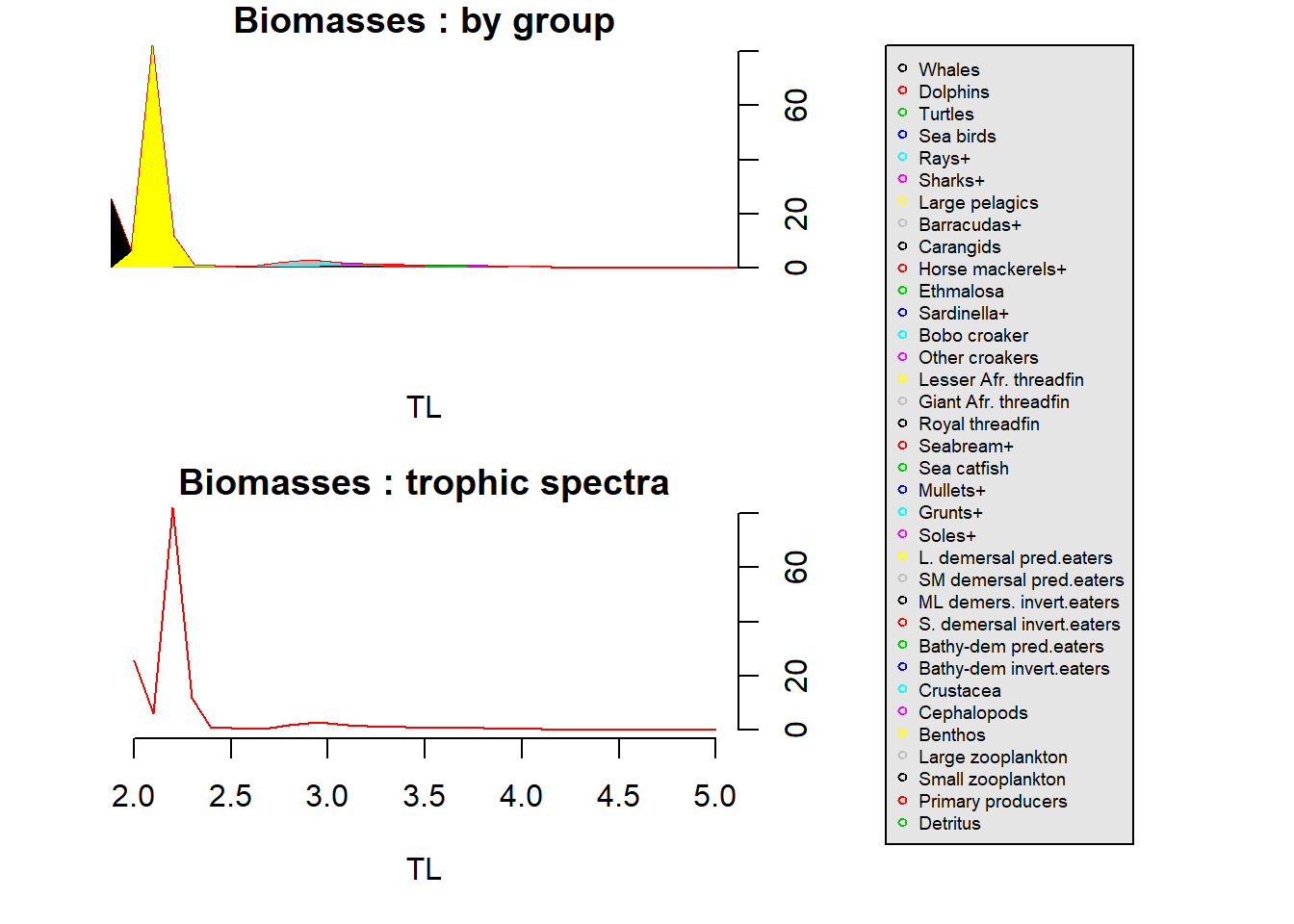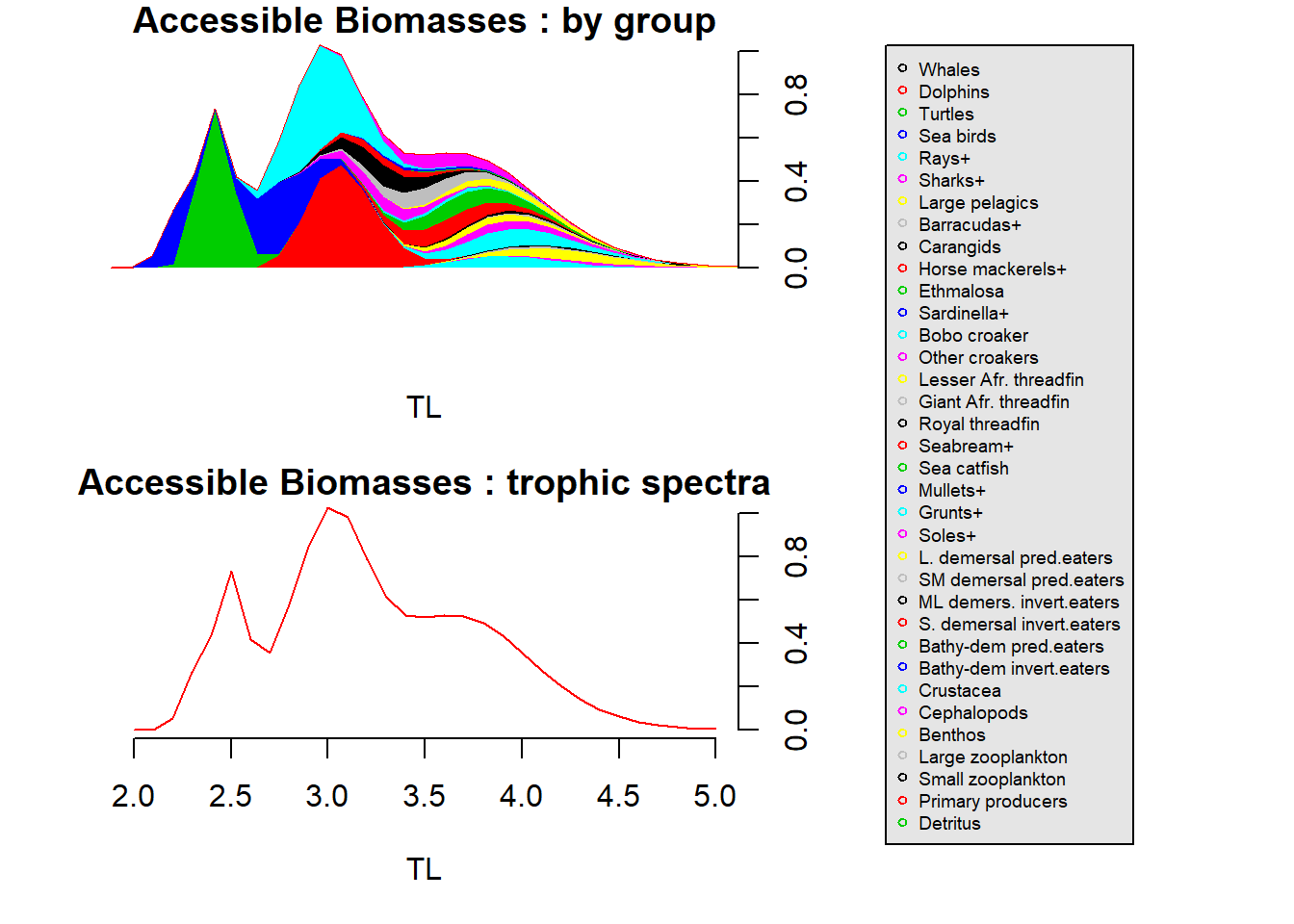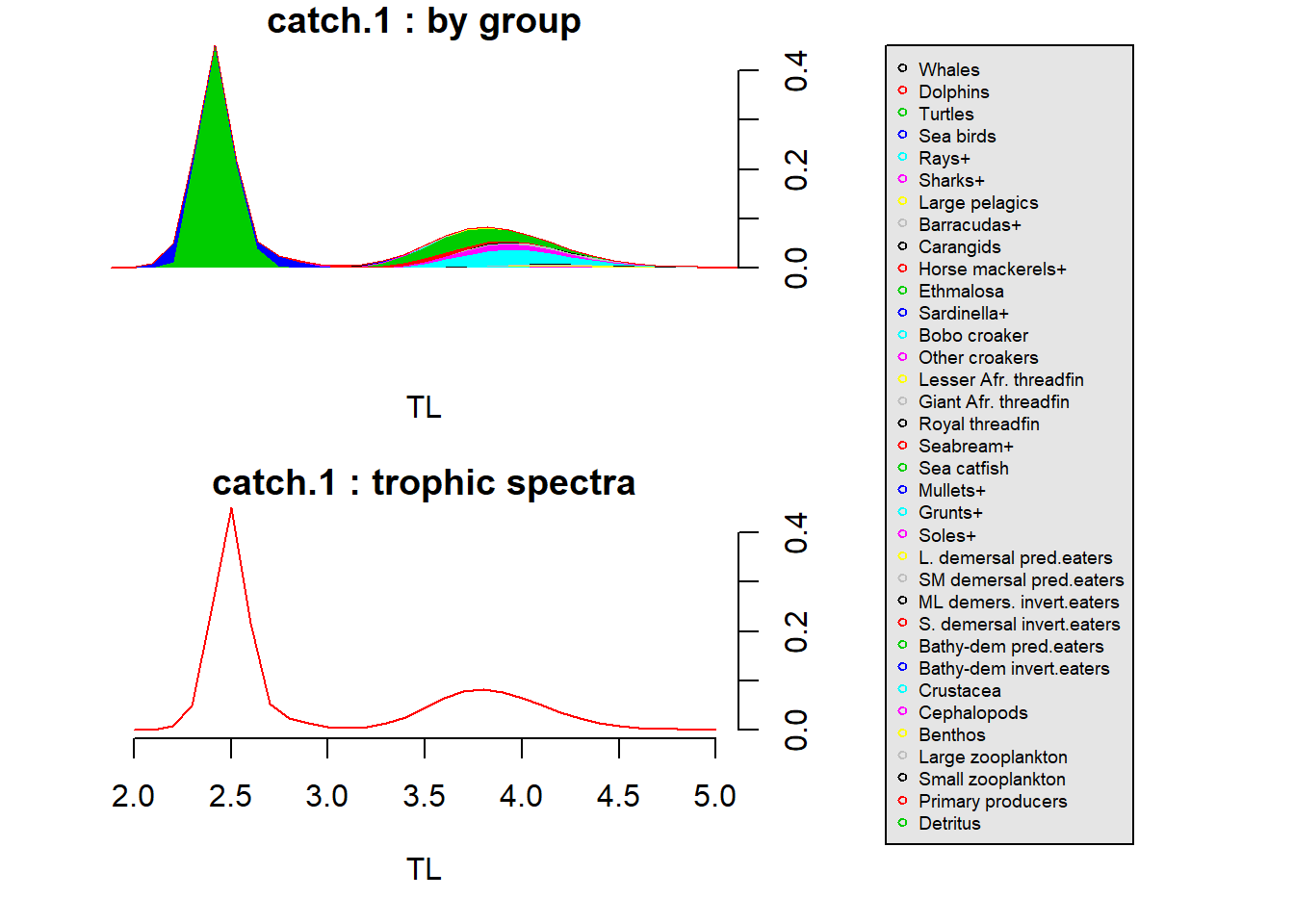EcoTroph Example

# 1. Installation of the EcoTroph package

The EcoTroph package version 1.6 is available on CRAN and can be installed by calling the install.packages function first and then load the package using the function library.

install.packages("EcoTroph")
library(EcoTroph)

# 2. Data import and validation

The EcoTroph package requires an input data table containing the following columns: 1) the name of the group representing one or several species gathered together (group_name), 2) the trophic level of the group (TL), 3) the biomass of the group (biomass), 4) the production over biomass ratio or P/B (prod), 5) the catch per fleet of the group (catch.1) and 6) the ratio of the group biomass that would be caught assuming an infinite fishing pressure (accessibility). These parameters generally come from an Ecopath model but can also be independent. Use catch.1, catch.2, catch.x if you have to insert several fisheries. Another column can be added containing the omnivory index of each group (OI). This index is used in the create.smooth function, which we will see in section 3.1.

Your data table should have any of the common formats (e.g. excel, csv, txt) and can be loaded into R using, e.g., the functions read.table or read.csv.
Below you find an example containing the first six rows of a suitable data frame. The example is based on the ecopath model ecopath_guinee (Gascuel et al., 2005; Gascuel et al., 2011) and is included in the package:

data("ecopath_guinee")          # load the example data
kable(head(ecopath_guinee))     # access the first six rows of the data.frame
group_name TL biomass prod catch.1 catch.2 accessibility OI
Whales 4.01 0.03090 0.020 0.000 0.000 0.0 0.059
Dolphins 4.48 0.04330 0.070 0.000 0.000 0.0 0.331
Turtles 2.19 0.02960 0.150 0.000 0.000 0.0 0.338
Sea birds 3.81 0.00128 0.300 0.000 0.000 0.0 0.353
Rays+ 3.97 0.38600 0.363 0.012 0.024 0.9 0.329
Sharks+ 4.31 0.10500 0.410 0.007 0.003 0.8 0.633

$$\color{red}{\text{Note, that the row names have to be specified exactly as above}}$$: group_name, TL, biomass, prod, catch.1, accessibility, OI (optional).

You can check the compatibility of your input data table with the EcoTroph package, using the check.table function:

check.table(ecopath_guinee)

If a message appears, read it carefully and correct the dataset in view of the comments. $$\color{red}{\text{No NA is accepted in the inputs}}$$, a yield column has to be entered with 0-values if no catches are registered. Yield and the P/B (prod) of detritus groups have to be set to 0.

# 3. Implementation of the EcoTroph approach

## 3.1. Creation of the smooth function

The smooth function creates a table enabling the conversion of data referring to specific taxons or functional group’s (e.g. Ecopath trophic groups) into data referring to trophic classes. The major assumption of this function is that the distributions of the trophic groups’ biomass, yield, etc. around their mean trophic level follow a lognormal distribution. The lognormal is defined by a mean (the mean trophic level of the group) and a standard deviation (sigmaLN) which is a measure of the trophic level variability within the group. The create.smooth function contains the following arguments:

$$\color{slategrey}{\text{create.smooth(tab_input,smooth_type=NULL,sigmaLN_cst=NULL,pas=NULL,shift=NULL,smooth_param=NULL)}}$$
• tab_input is the input data table
• pas defines the splitting of the trophic classes and has by default a value of 0.1.
• the argument smooth_type defines the form of the standard deviation (sigmaLN) for the lognormal distribution. Three options are implemented:
1. If smooth_type=1 (default), sigmaLN is constant. This constant sigmaLN, corresponding to the argument sigmaLN_cst in the function, has by default a value of 0.12, with a shift value (shift) of 1.8 by default.
2. If smooth_type=2, sigmaLN=smooth_paramln$$\times(TL-0.05)$$. The argument smooth_param of this formula defines the slope of the log-linear trophic level variability increase around the mean trophic level of the group. By default, smooth_param=0.07 and shift=0.95 (no need to change the shift value in the function, let shift=NULL; it will be automatically adjusted at 0.95 for smooth_type=2. Same for the argument smooth_param).
3. If smooth_type=3, sigmaLN for each group is equal to the omnivory index calculated by Ecopath. A warning message will appear if OI’s are equal to 0, they will be automatically changed for a value of 0.01. The argument shift is by default equal to 0 (no need to change the shift value in the function; let shift=NULL, it will be automatically adjusted at 0 for smooth_type=3).

The create.smooth function returns a table of the distribution of each mean trophic level within trophic classes. This enables the construction of trophic spectra.

create.smooth(ecopath_guinee)                      # default choice, sigmaLN
create.smooth(ecopath_guinee, smooth_type = 2)     # sigmaLN=smooth_param*ln(TL-0.5)
create.smooth(ecopath_guinee, smooth_type = 3)     # sigmaLN=ecopath_guinee\$OI

We also included the possibility to graphically explore the outputs of the different EcoTroph functions. To plot the output of the create.smooth function, simply call the plot function. For smooth_type 2:

plot(create.smooth(ecopath_guinee, smooth_type=2))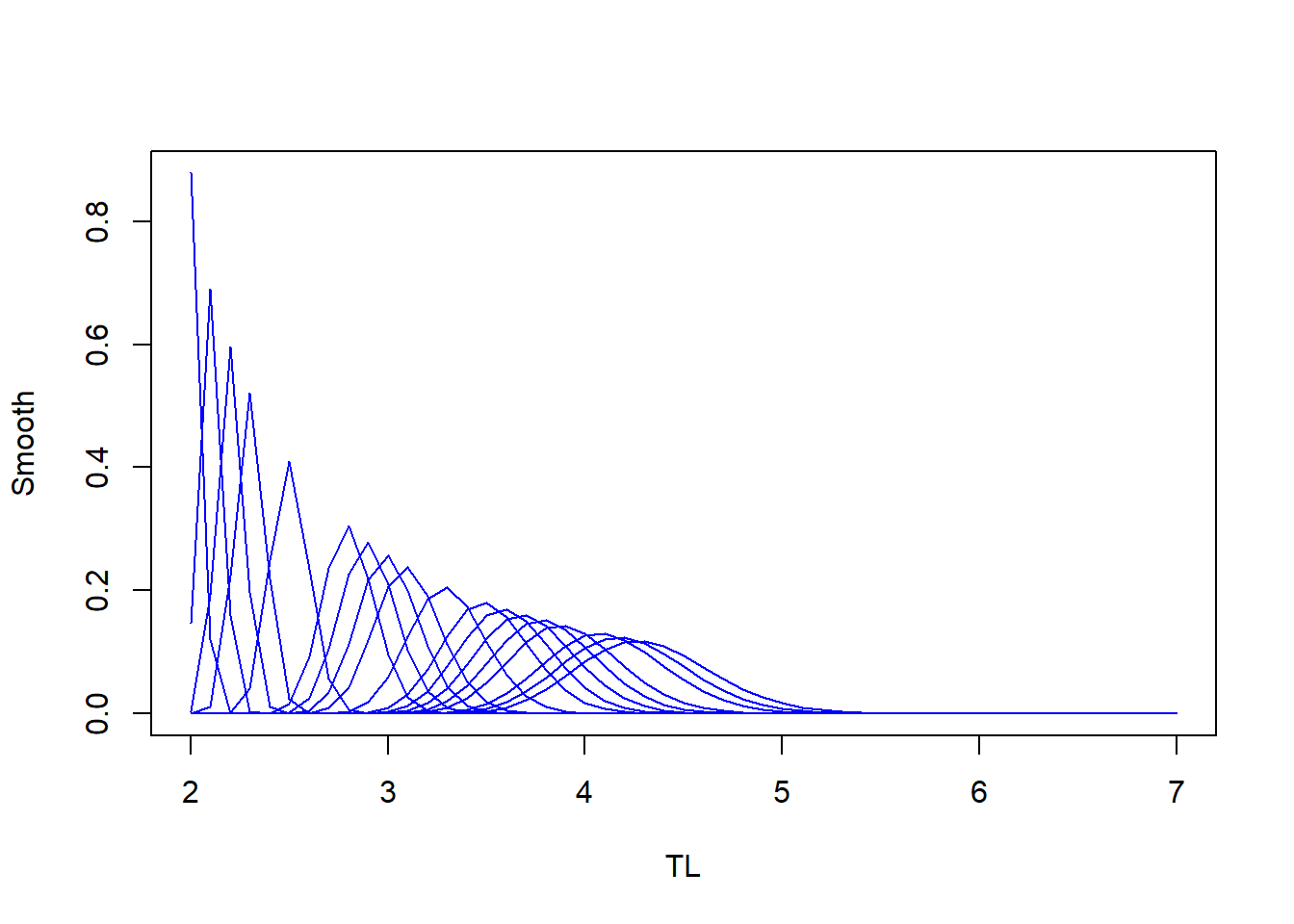For smooth_type 3

plot(create.smooth(ecopath_guinee, smooth_type=3))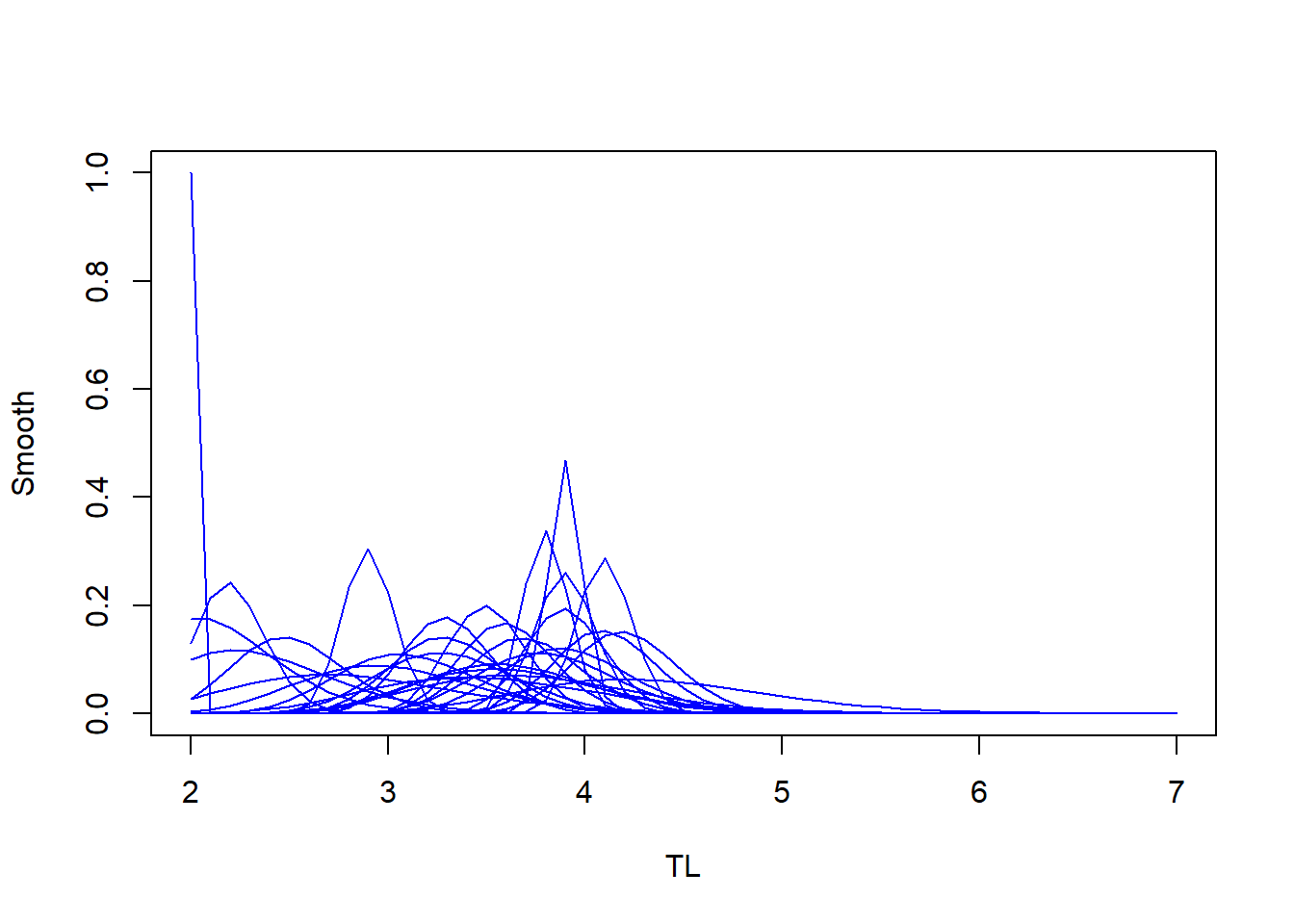## 3.2. Data Transposition

The Transpose function uses the output of the create.smooth function to convert the biomass, catches and P/B (prod) values referring to specific taxons or functional groups into data referring to trophic classes. The Transpose function, distributes these variables continuously along the trophic classes. Transpose contains the following arguments:

$$\color{slategrey}{\text{Transpose(tab_smooth, tab_input, column)}}$$
• tab_smooth is the table returned by the create.smooth function
• tab_input is the input data table
• column is the column (e.g. biomass or catch) you want to distribute by trophic class
Transpose(create.smooth(ecopath_guinee), ecopath_guinee, "biomass")     # transpose of the biomass column
Transpose(create.smooth(ecopath_guinee), ecopath_guinee, "catch.1")     # transpose of the catch.1 column

Results are displayed graphically calling the plot function on the table returned by the Transpose function. It returns the principal plots according to the selected column, in particular a plot by group and the associated trophic spectra: biomass trophic spectra (‘biomass’ column), catch trophic spectra (‘catch’ column), P/B trophic spectra (‘prod’ column). Results are displayed graphically calling the plot function on the table returned by the Transpose function.

$$\color{slategrey}{\text{plot(x, scale = NULL, title = NULL, legend.cex = NULL)}}$$
• x is the table returned by the Transpose function
• scale specifies whether or not the scale of the y-axis is logarithmic (scale = “log”), by default the scale is linear.
• title is an argument used to display the title of the plot.
• legend.cex controls the size of the legend text (default = 0.8).
plot(Transpose(create.smooth(ecopath_guinee), ecopath_guinee, "biomass"), title="biomass", log, legend.cex=0.6) # log scale for the biomasses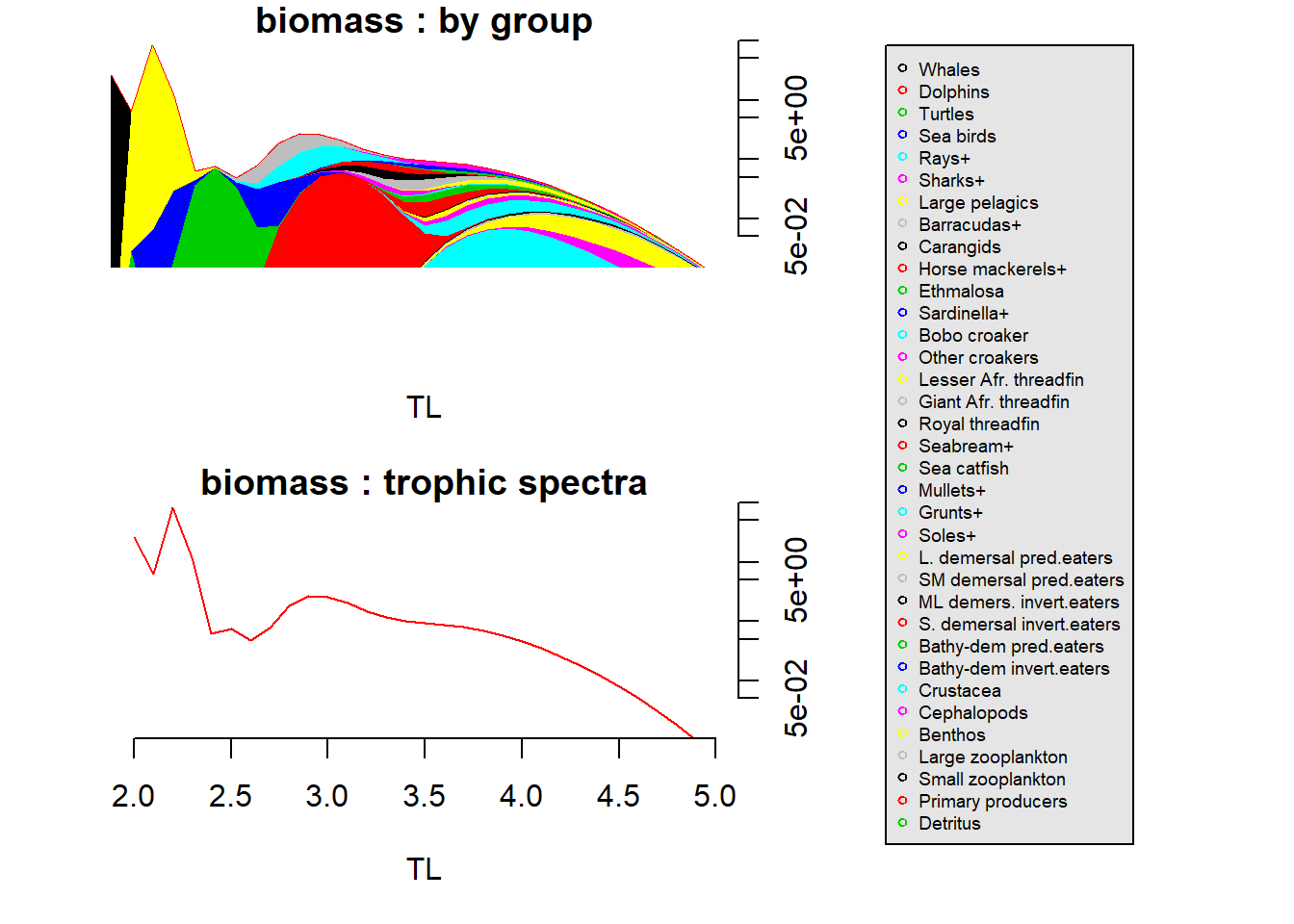plot(Transpose(create.smooth(ecopath_guinee),ecopath_guinee,"catch.1"),title="Small scale fishery", legend.cex=0.6) # insertion of a title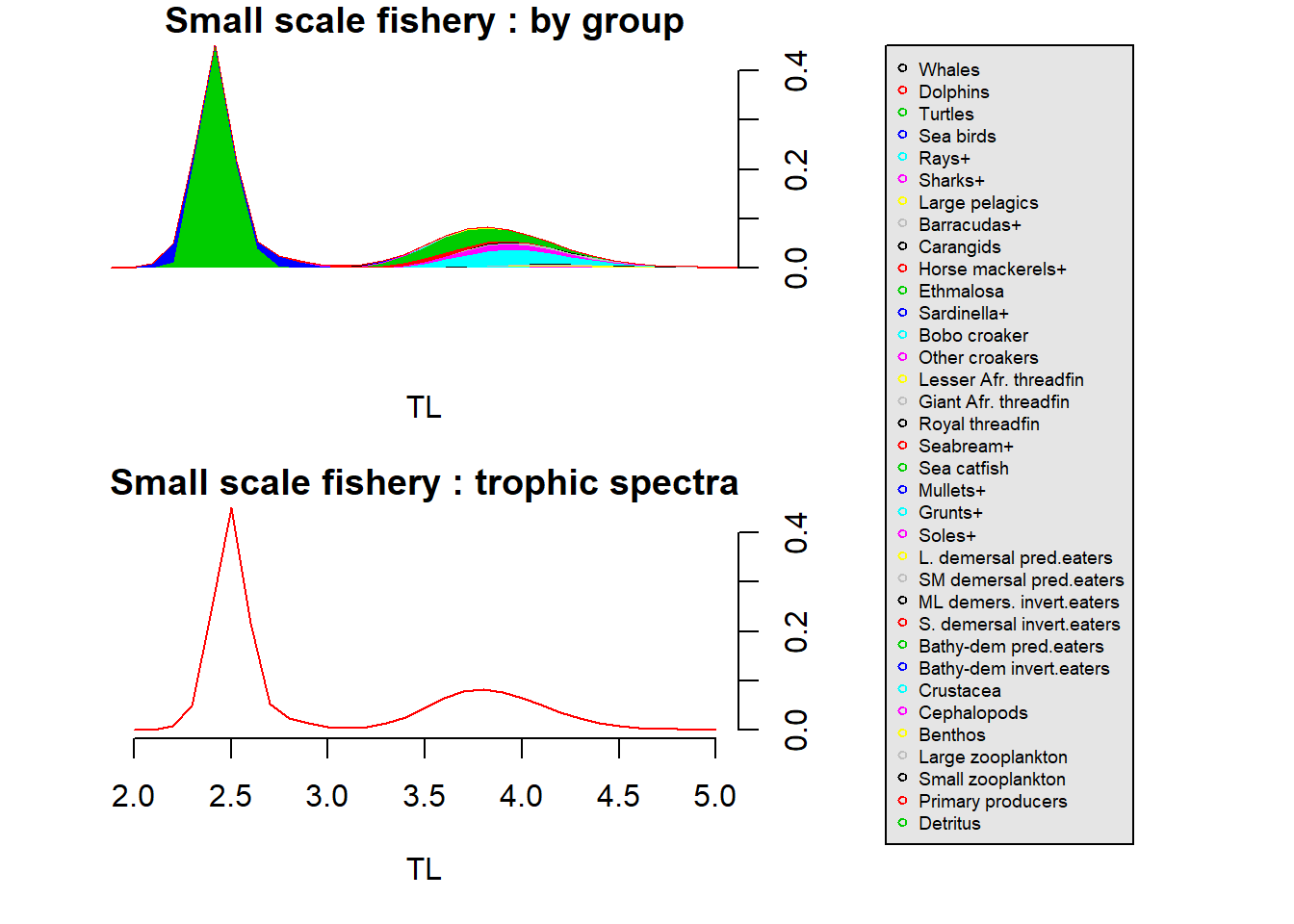## 3.3. ET.Main table construction

The create.ETmain function returns a list with 1) a summary table, called ET_Main, containing the principal variables by trophic class; and 2) some intermediate tables: biomass (biomass), accessible biomass (biomass_acc), P/B (prod), accessible P/B (prod_acc), the distribution of each mean trophic level within trophic classes (tab_smooth) and the catch tables (Y). This function can be called directly on the data input table. There is no need of the previous steps (3.1 and 3.2). However, we highly recommend you to follow them for a proper understanding of the EcoTroph model. The create.ETmain function contains the following arguments:

$$\color{slategrey}{\text{create.ETmain(ecopath,smooth_type=NULL,sigmaLN_cst=NULL,pas=NULL,shift=NULL,smooth_param=NULL)}}$$
• ecopath is the input data table
• other arguments are arguments of the create.smooth function (3.1.)
create.ETmain(ecopath_guinee)                              # constant sigmaLN
create.ETmain(ecopath_guinee,smooth_type=2)                # sigmaLN=smooth_param*ln(TL-0.05) (cf. 2.1)

Results are displayed graphically calling the plot function on the table returned by the creat.ETmain function. Different plots are created, for instances the biomass trophic spectra, the accessible biomass trophic spectra and the catch trophic spectra. The plot function called on the output of the create.ETmain function, takes the following arguments:

$$\color{slategrey}{\text{plot(x, scale1 = NULL, scale2 = NULL, scale3 = NULL, legend.cex = NULL, ask = interactive)}}$$
• x is the table returned by the create.ETmain function
• scale1, scale2 and scale3 specify whether or not the scale of the y-axis in the biomass, accessible biomass or catch plots is logarithmic (scale = “log”) or not (default is linear).
• legend.cex controls the size of the legend text (default = 0.8).
• ask is an argument used to control the display of each graph. The default value is interactive.
plot(create.ETmain(ecopath_guinee),legend.cex = 0.6)